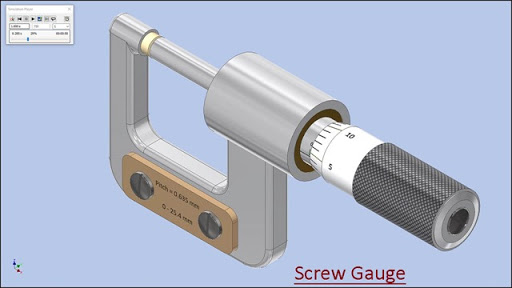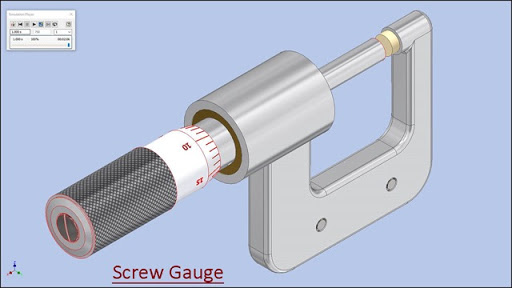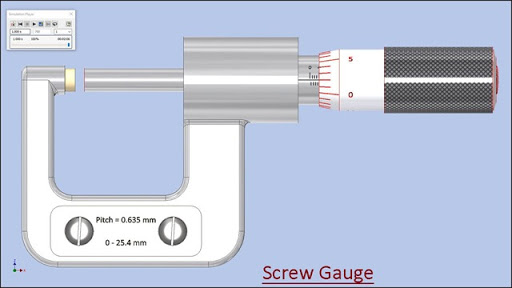## Friday, September 28, 2018

### Modelling of 'Screw Gauge' with Dynamic Simulation (Volume-2) Autodesk Inventor 2018 Tutorial#### Serial No. 305-B.

Modelling of 'Screw Gauge' with Dynamic Simulation (Volume-2) Autodesk Inventor 2018 Tutorial(Starting of the model is designed in Volume-1)Click the following link to get the model file: - https://autode.sk/2DLeKjg

#### Procedure of reading of Screw Gauge

Total number of divisions imprinted on Circular scale (in one round) = 25

Total number of divisions imprinted on Linear scale = 40

Pitch of Linear scale = total length of Linear scale/total number of divisions imprinted on Linear scale
= 25.4mm/40 = 0.635mm

Least count of Circular scale = Pitch of Linear scale/total number of divisions imprinted on Circular scale
= 0.635mm/25 = 0.0254mm

Reading of Linear scale = Pitch of Linear scale x Number of divisions opened on Linear scale
= 0.635mm x 4 = 2.54mm

Reading of Circular scale = Least count of Circular scale x Number of divisions
ahead of central line of Linear scale
= 0.0254mm x 2 = 0.0508mm

Total Reading = Reading of Linear scale + Reading of Circular scale
= 2.54mm + 0.0508mm = 2.5908mm